# Tate conjectures

(diff) ← Older revision | Latest revision (diff) | Newer revision → (diff)

Conjectures expressed by J. Tate (see ) and describing relations between Diophantine and algebro-geometric properties of an algebraic variety.

Conjecture 1. If the fieldis finitely generated over its prime subfield, ifis a smooth projective variety over, ifis a prime number different from the characteristic of the field, if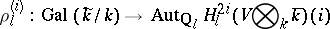is the natural-adic representation, and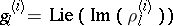, then the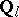-space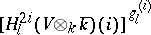, the space of elements of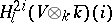annihilated by, is generated by the homology classes of algebraic cycles of codimensionon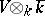(cf. also Algebraic cycle).

Conjecture 2. The rank of the group of classes of algebraic cycles of codimensiononmodulo homology equivalence coincides with the order of the pole of the functionat the point.

These conjectures were verified for a large number of particular cases; restrictions are imposed both on the fieldand on the variety.

How to Cite This Entry:
Tate conjectures. Encyclopedia of Mathematics. URL: http://encyclopediaofmath.org/index.php?title=Tate_conjectures&oldid=12224
This article was adapted from an original article by S.G. Tankeev (originator), which appeared in Encyclopedia of Mathematics - ISBN 1402006098. See original article